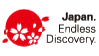# Support

## title(h2)

This is the sample text. This is the sample text. This is the sample text. This is the sample text. This is the sample text. This is the sample text. This is the sample text. This is the sample text.
This is the sample text. This is the sample text. This is the sample text. This is the sample text. This is the sample text. This is the sample text. This is the sample text. This is the sample text.